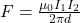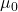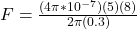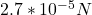## Two long parallel wires that are 0.30 m apart carry currents of 5.0 A and 8.0 A in the opposite direction. Find the magnitude of the force p

Question

Two long parallel wires that are 0.30 m apart carry currents of 5.0 A and 8.0 A in the opposite direction. Find the magnitude of the force per unit length that each wire exerts on the other wire and indicate if the force is attractive or repulsive.

in progress 0
6 months 2021-07-22T15:20:40+00:00 1 Answers 25 views 0

1. To solve this problem we will apply the concepts related to electromagnetic force. This force is defined as the product between the free space permeability constant, the product of the two currents found, at the rate of the distance that separates them. In this way mathematically it can be given as,Here,= Permeability of free space

I = Each current

d = Distance

Replacing with our values,Therefore the force is